In this lab we will review concepts of community detection and how to represent our network in a map.

First we will load the data and the libraries we will be using. Remember that on the previous lab we exported a data set at the end, we will continue using this objects.

# If you are starting a new session, load the files and libraries again
# libraries we will use.
library(dplyr) # Data manipulation
library(sf) # Spatial data manipulation
library(tidygraph) # Network manipulation and analysis
library(purrr) # for data manipulation
library(ggraph) # for network visualization
library(ggpubr) # for arranging plots

# 1 Community detection

In this section we will use different algorithms to identify communities in our network.

# First we need to simplify the network
c <- net %E>%  # we call our network and activate the edges
mutate(N = as.integer(1)) %>%  # create a variable for the number of movements (each row is 1 movement)
convert(to_simple) %E>%  # now we will convert it to a simple network
mutate(weight = map_int(.orig_data, ~.x %>% pull(N) %>% sum())) %N>%  # We have to sum all the repeated movements
mutate(walktrap = factor(group_walktrap(weights = weight)), # use the walktrap algorithm for community detection
infomap = factor(group_infomap(weights = weight))) # use the infomap for community detection

Then we will create an empty list to fill with plots and compare the different algorithms.

# Create the empty list
CP <- list()
# Make a plot for the edges only
pc <- c %>% # our simplified network
ggraph(layout = 'nicely') + # call the ggraph function
theme(legend.position = 'bottom') # set the legend position to bottom

CP[['Infomap']] <- pc + # We call our plot with only the edges
geom_node_point(aes(col = infomap), size = 2) + # we add the nodes
labs(title = 'Infomap') # title of our plot

CP[['Walktrap']] <- pc +
geom_node_point(aes(col = walktrap), size = 2) +
labs(title = 'Walktrap')

# We arrange our plots in a single figure
ggarrange(plotlist = CP)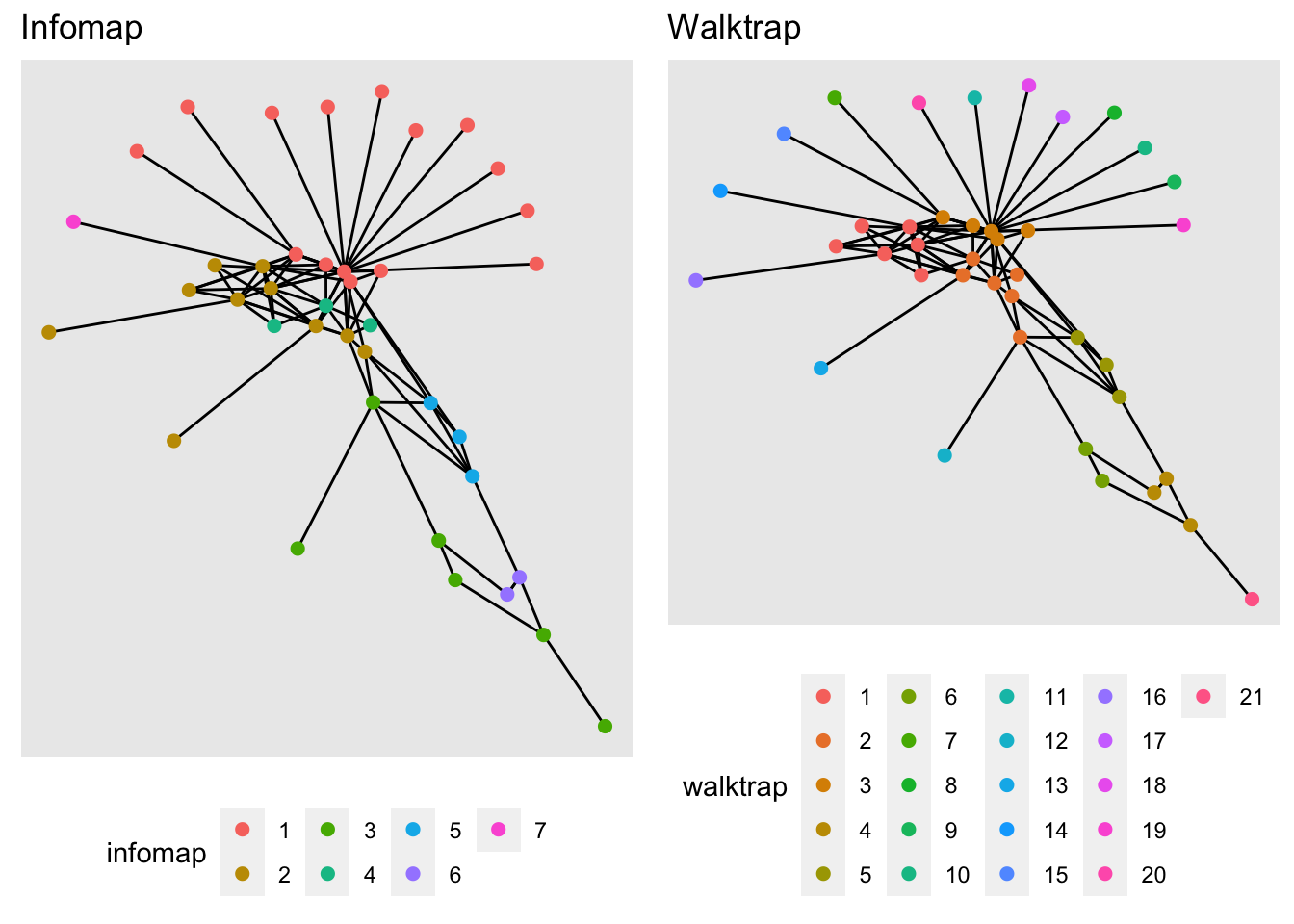Excercise: Now increase the number of steps in the walk trap algorithm, what happens when we increase the number of steps? What do you think would be the optimal number of steps for this example

# 2 Spatial representation of the network

Now we will use the network we created and the spatial location of our farms to see the movements on a map.
We will be using the sf package to manipulate the spatial objects, and the ggplot2 package for visualization.
In the STNet package there is a spatial polygons data, which includes the counties in the state of Iowa.

# Loading the packages
library(sf) # Package for spatial objects
library(ggplot2) # package for plots

# We load the spatial object from the package STNet
iowa <- st_read(system.file("data/Io.shp", package = "STNet"))
## Reading layer Io' from data source
##   /Library/Frameworks/R.framework/Versions/4.1/Resources/library/STNet/data/Io.shp'
##   using driver ESRI Shapefile'
## Simple feature collection with 99 features and 2 fields
## Geometry type: POLYGON
## Dimension:     XY
## Bounding box:  xmin: -96.63567 ymin: 40.37454 xmax: -90.13931 ymax: 43.50465
## Geodetic CRS:  WGS 84
# plot map using sf
plot(iowa$geometry)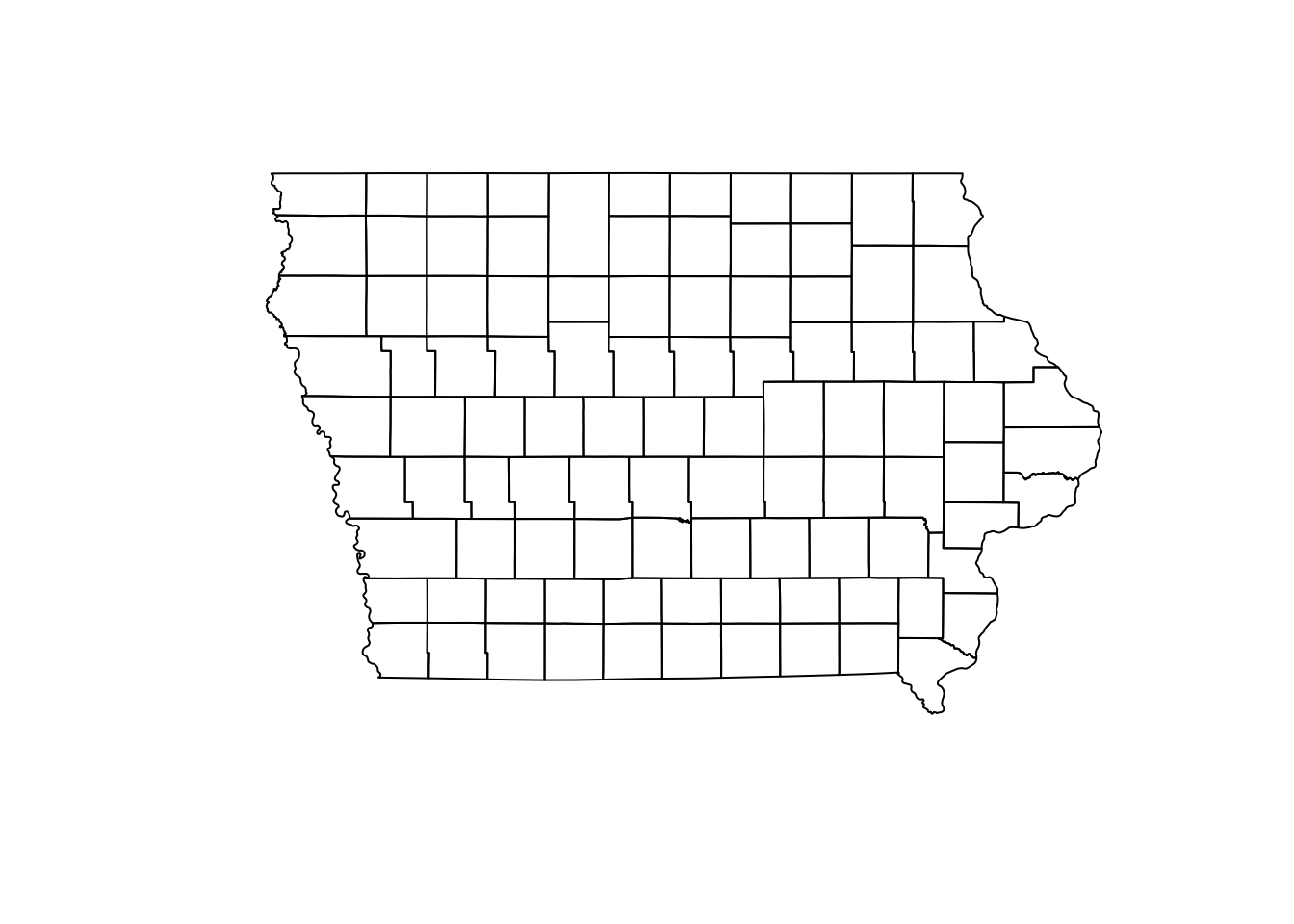Next we will transform the nodes as a spatial points object, for this we use the function st_to_sf() and we need to specify the names of the columns that have the spatial coordinates. NodeSp <- node %>% # This is our node data.frame st_as_sf(coords = c("long", "lat"), # Variables for the coordinates crs = st_crs(iowa)) # This is the CRS we are using ## 2.1 Plotting our map One of the nice things of ggplot is that we can create a map and store it in an object and later we can keep adding layers to this map. So first we will create a map of the state. map <- ggplot() + geom_sf(data = iowa, # name of the spatial dataset color="grey20", # color of the shape border fill="white", # fill of the shape size=0.4) + # width of the border theme_void() # This is a theme form ggplot ## 2.2 Plot the nodes Once we have the base map of the state, we can add the spatial points data we created previously. We can specify the size of the points using a variable. map + geom_sf(data = NodeSp, # name of our data aes(color = farm_type, # we color the nodes by farm type size = indegree)) + ggtitle("Farms and their indegree") # the title of our plot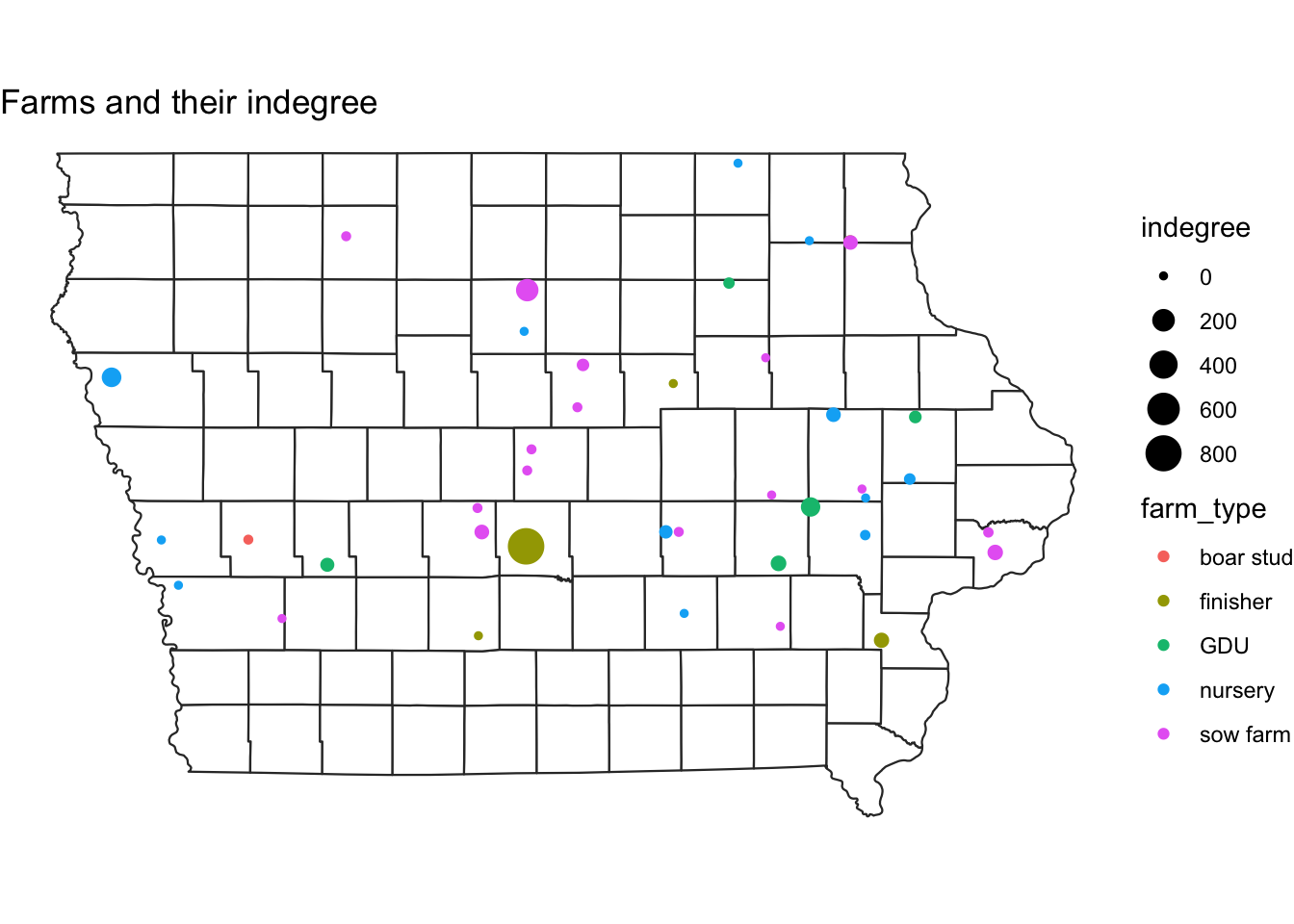Excercise: Make the same plot, but make the size of the nodes relative to outdegree ## 2.3 Adding the euclidean contacts In the previous lab we calculated the euclidean distance between each pair of farms involved in a movement. Here we will visualize those movements. # The function geom_segment adds a straight line between two coordinates: map + geom_segment(data=edge, aes(x=O_Long, y=O_Lat, # this is where the line starts xend=D_Long, yend=D_Lat)) # this is where it ends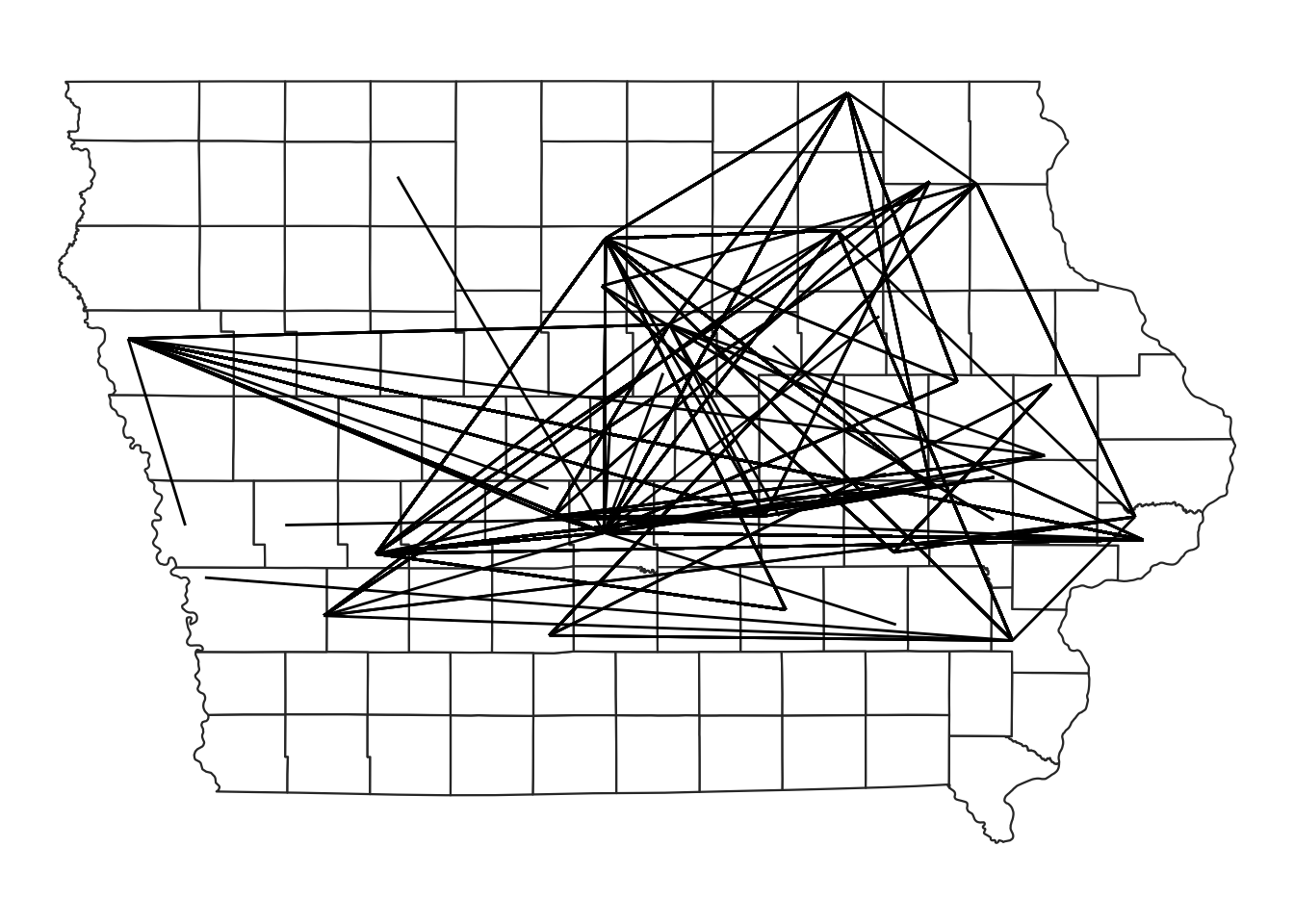# We can add the information of the type of movement to change the color of the line and the number of animals for the transparency map + geom_segment(data=edge, aes(x=O_Long, y=O_Lat, xend=D_Long, yend=D_Lat, color=type_orig, alpha = pigs.moved))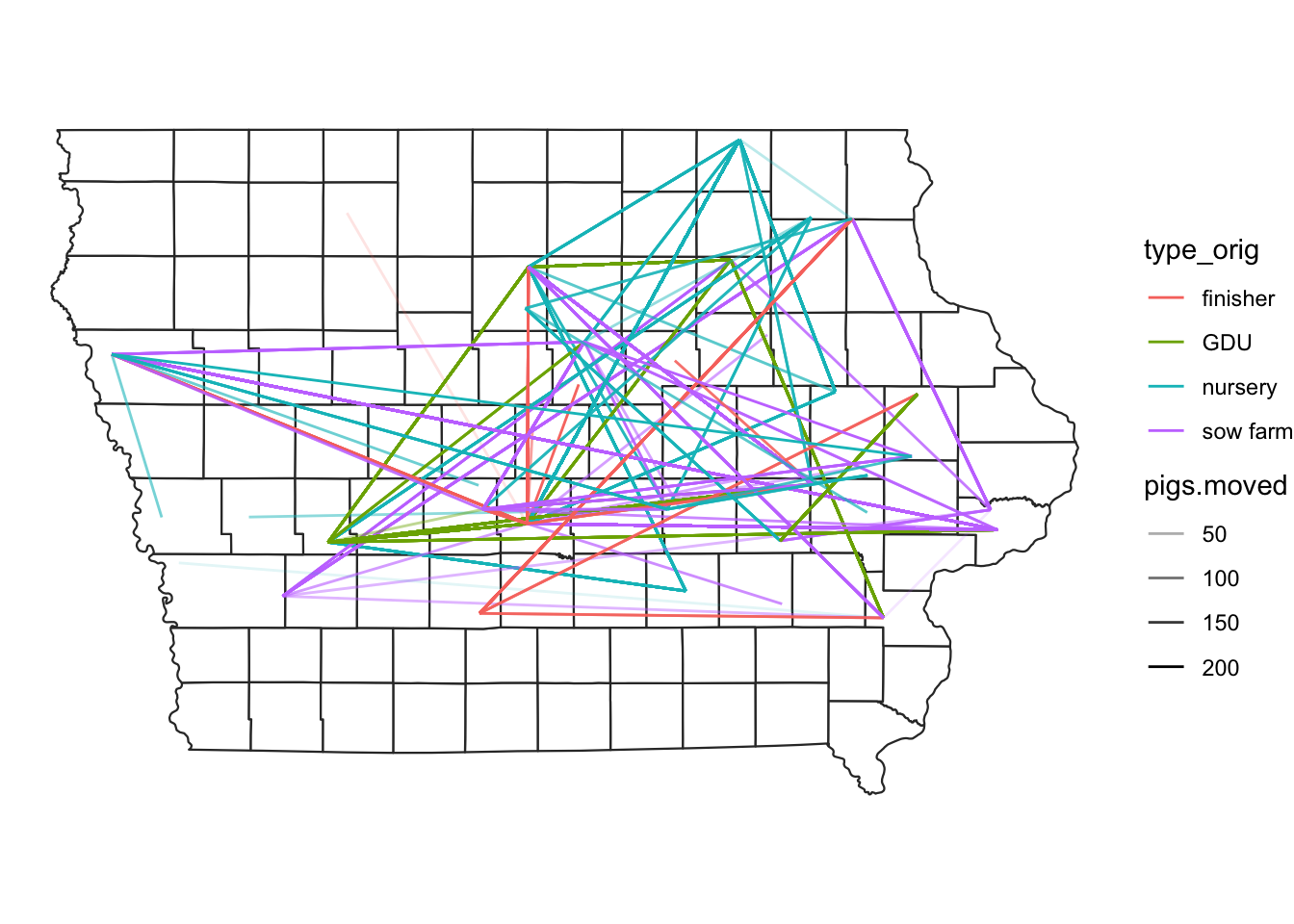## 2.4 Putting everything together Now we will add both the farm locations and the direction of the movements between the farms on a map. #plot nodes & edges - add both commands geom_segment and geom_point# map + geom_segment(data=edge, aes(x=O_Long, y=O_Lat, xend=D_Long, yend=D_Lat, alpha = pigs.moved), show.legend=F) + geom_sf(data = NodeSp, aes(color = farm_type, size = indegree), show.legend = "point")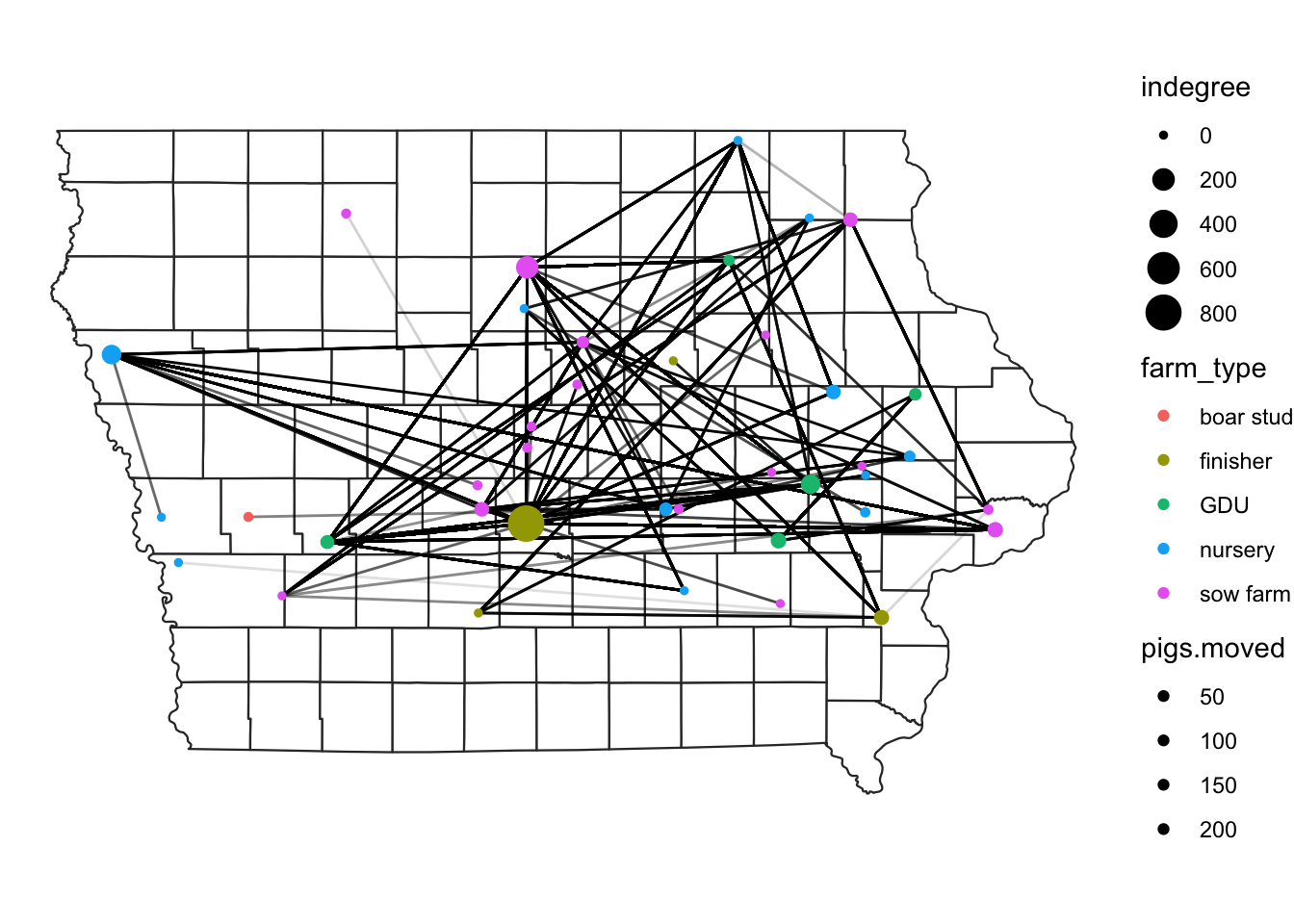## 2.5 Subsetting the data Sometimes we are interested in a particular type of movements. We can subset this using the dplyr functions such as filter(). In the next plot we will select only the movements that comes from sow farms. # plot movements from sow farms only map + edge %>% filter(type_orig == "sow farm") %>% geom_segment(data = ., aes(x=O_Long, y=O_Lat, xend=D_Long, yend=D_Lat, color = type_orig), show.legend = F) + geom_sf(data = NodeSp, aes(color = farm_type), size=3, show.legend = "point")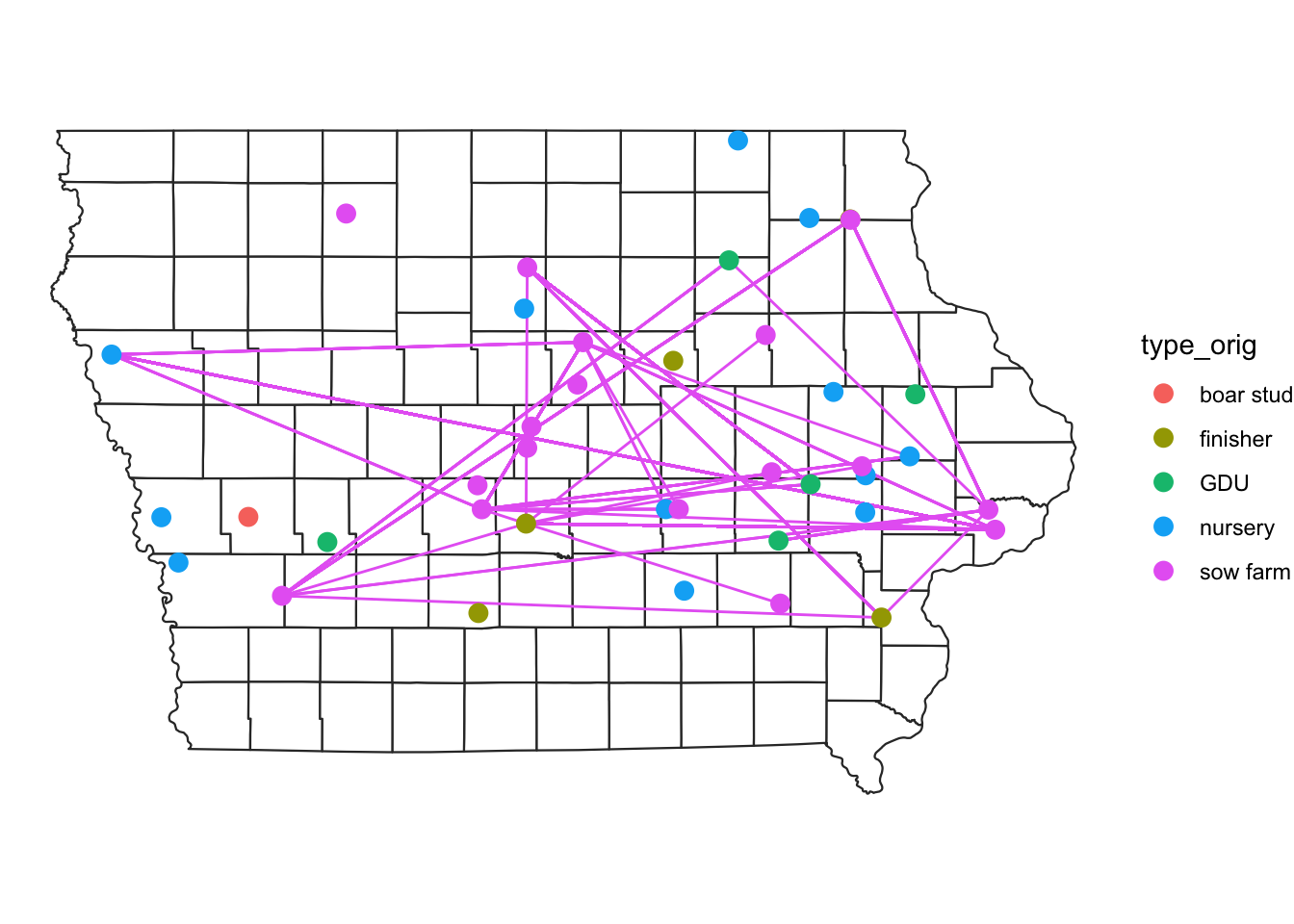We can be even more specific and filter the movements from sow farms that are directed to GDU. We will also add at the end the function ggplotly() from the package plotly to obtain a map were we can zoom and hover over some features to obtain more information. # We store the map of movements between GDU to sow farm m <- map + edge %>% filter(type_orig == 'GDU', type_dest == "sow farm") %>% geom_segment(data = ., aes(x=O_Long, y=O_Lat, xend=D_Long, yend=D_Lat, color = type_orig), show.legend = F) + geom_sf(data = NodeSp, aes(color = farm_type), size=3, show.legend = "point") + ggtitle("GDU to Sow farm Movments") # We use the function from plotly to transform our map into n interactive map. library(plotly) ggplotly(m) # 3 Kernel density map Like we just saw, visualizing the movements can be challenging, one approach to do this is using a kernel density map. The idea behind this is to extrapolate values in a continuous surface, but here we are just interested in the visualization of the values, not so much in the interpolation of our values. We will use the package KernSmooth for this, so make sure you ahve it installed. First we will define a function to automate the process: library(KernSmooth) library(raster) # we will create a function to create a density raster: processRaster <- function(x, b, shp, res = c(200, 200)) { est <- bkde2D( # we use the function bkde2D to obtain our values x, # This will be our dataset bandwidth = c(b, b), # The bandwidth we define gridsize = res, # the resolution level we want range.x = list(extent(shp)[c(1, 2)], extent(shp)[c(3:4)]) ) # Add the results to a raster r <- raster(list( x = est$x1,
y = est$x2, z = est$fhat
)) %>%
projection<-(st_crs(iowa)) %>% # set the CRS
extent<-(extent(iowa)) %>% # set the extent
crop(., iowa) %>% # crop the raster to the area
mask(., iowa) # crop the raster to the stat shape

return(r)
}

Now lets use our function for the data.

# Obtain the estimated kernel with bandwidth 2km
Erc <- processRaster(x = edge[,c("O_Long", "O_Lat")], # we want the outgoing only
b = 2, # we choose a bandwidth of 2
shp = iowa) # we set our extent

# plot the raster and the map
plot(Erc)
plot(iowa$geometry, col=NA, border = "grey80", add = T)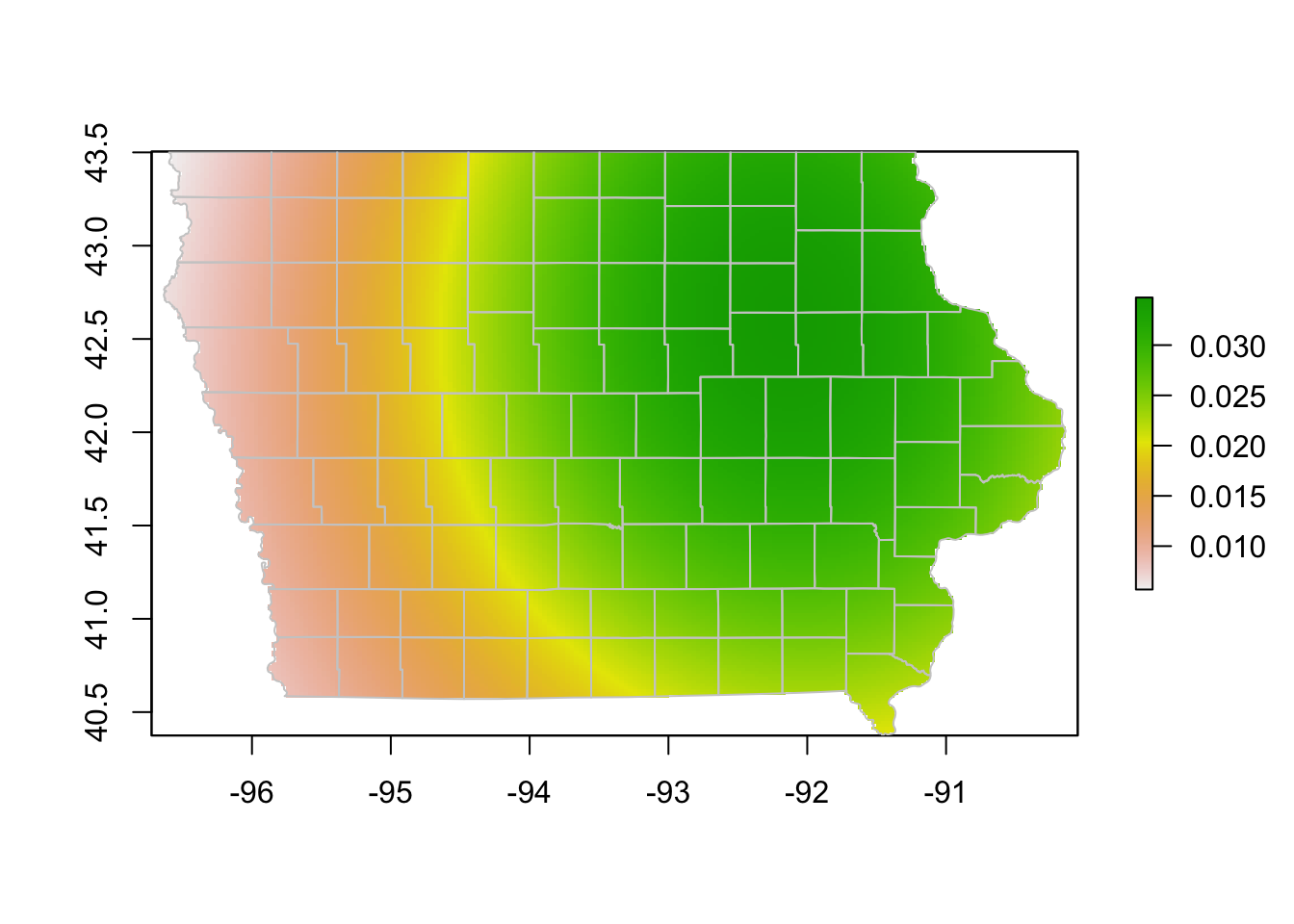We might have used a very large bandwidth in the previous plot, lets try with a smaller size. # Using a different bandwidth Erc <- processRaster(x = edge[,c("O_Long", "O_Lat")], b = 0.1, shp = iowa) # plot the raster and the map plot(Erc) plot(iowa$geometry, col=NA, border = "grey80", add = T)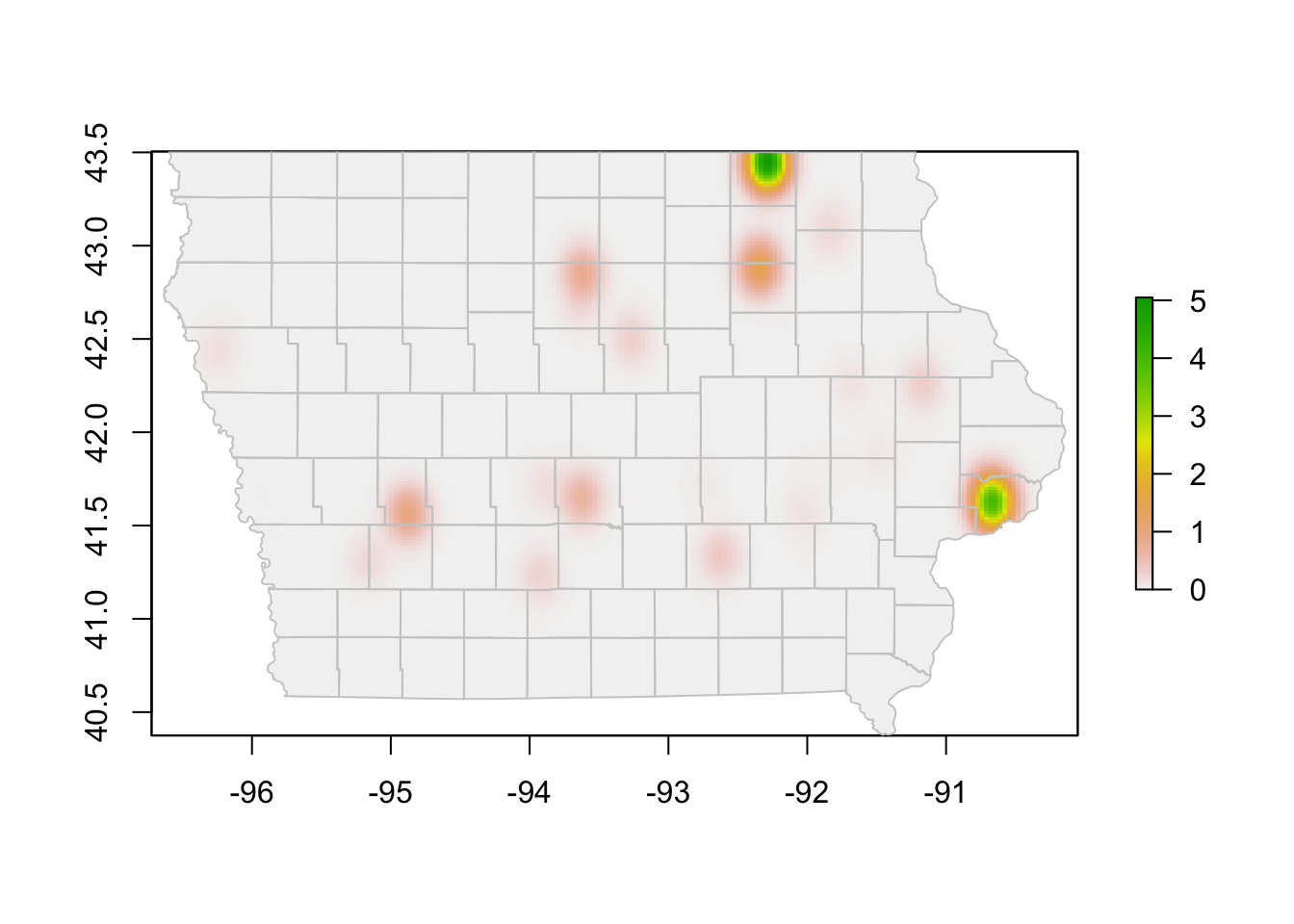Exercise: Create a kernel density map for the incoming movements.

# 4 More on interactive maps.

If you are interested in more about interactive maps plotly also has option for using background maps, but for this you need to get a public Mapbox access token, which is free, but requires registration. Some great resources for more information:

Here I provide an example of the kind of maps you can get using mapbox, but this This code will not run unless you use your own API Key

# Sys.setenv('MAPBOX_TOKEN' = yourKey)

plot_mapbox() %>%
data = group_by(edge, id_orig, id_dest),
x = ~O_Long, xend = ~D_Long,
y = ~O_Lat, yend = ~D_Lat,
alpha = 0.1, size = I(1), hoverinfo = "none"
)   %>%
))`